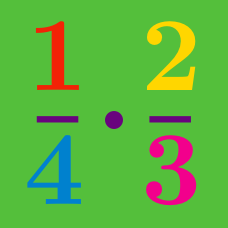Basic Mathematics

# Fractions

#### Challenge Quizzes

Evaluate $\frac{3}{4} + \frac{1}{4} + \frac{3}{4}.$

If the expression $\frac{5}{3}+\frac{3}{4}$ is written in simplest form and as an improper fraction, what is the value of the numerator?

Evaluate $5\frac{2}{3} - 1\frac{1}{3} - 2\frac{2}{3}.$

Evaluate $\frac{13}{2}-\frac{5}{3}+\frac{3}{2}-\frac{7}{3}.$

Which of the following is equal to

$\frac{14}{3}+\frac{3}{6} ?$

×